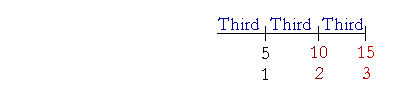S k i l l
i n
A R I T H M E T I C

Lesson 17  Section 2

PROPORTIONS

What is taught in most textbooks these days as ratio and proportion, is not. It is algebra. The student is taught to represent a ratio as a fraction, write the letter x for the unknown term, cross-multiply and solve an equation. That is an algebraic calculator.

A ratio requires understanding. It has meanng and is not merely symbolic. Because of that, the topic of ratio and proportion is educational.

 3. What is a proportion? A statement that two ratios are the same.

Example 1.   5 is to 15  as  8 is to 24.

That is a proportion because 5 is the third part of 15, just as 8 is the third part of 24.  (Lesson 15.)

A proportion involves four numbers -- four terms.  To explain why they are proportional we start with the first term and state its ratio to the second; then we state that the third term has that same ratio to the fourth.

Example 2.   Why is this a proportion?

16 is to 2  as  80 is to 10.

Answer.  This is a proportion because 16 is eight times 2, just as 80 is eight times 10.

Example 3.   Why is this a proportion?

10 is to 15  as  2 is to 3.Answer.  This is a proportion because 10 is two thirds of 15, just as 2 is two thirds of 3. (Lesson 15.)

Example 4.   Complete this proportion:

8 is to 32  as  9 is to ?

Solution.   8 is the fourth part of 32.  And 9 is the fourth part of 36.

The 1st term is to the 2nd  as  the 3rd is to the 4th.

Example 5.   Complete this proportion:

27 is to 3  as  ? is to 5

Solution.   27 is nine times 3.  And 45 is nine times 5.

If the student will speak and use sentences, the answer will be clear.

Example 6.   In each item below, what ratio has a to b?

(a simply means the first term; b means the second.)

a)  a is to b  as  1 is to 6.

b)  a is to b  as  10 is to 1.

a)  Since 1 is the sixth part of 6, then a is the sixth part of b.

b)  Since 10 is ten times 1, then a is ten times b.

Symbolic representation

The following is the traditional way to symbolize a proportion:

1 : 2 = 4 : 8.

"1 is to 2 as 4 is to 8."

Why is this a proportion?  Because 1 is half of 2, and 4 is half of 8.

Example 7.   Read this proportion, and complete it:

8 : 2 = 20 : ?

Answer.  "8 is to 2 as 20 is to what number?"

Now, what ratio has 8 to 2?

8 is four times 2.  And 20 is four times 5.

We say that we have solved that proportion. That is, given three terms, we have named the fourth.

Example 8.   Read this proportion and complete it:

7 : 21 = 4 : ?

Answer.  "7 is to 21 as 4 is to what number?"

What ratio has 7 to 21?

7 is the third part of 21.  And 4 is the third part of 12.

Example 9.   Complete this proportion:

2 : 3 = ? : 12

Answer.  "2 is to 3 as what number is to 12?"

Now, 2 is two thirds of 3.  What number is two thirds of 12?

"3 goes into 12 four times. Four times 2 is 8."

2 : 3 = 8 : 12.

In the next lesson we will see how to solve a proportion that looks like this:

5 : 7 = 20 : ?

At this point, please "turn" the page and do some Problems.

or

Continue on to the next Section.

Previous Section

1st Lesson on Parts of Natural Numbers